graph_tool.centrality - Centrality measures¶

This module includes centrality-related algorithms.

Summary¶

 pagerank Calculate the PageRank of each vertex. betweenness Calculate the betweenness centrality for each vertex and edge. central_point_dominance Calculate the central point dominance of the graph, given the betweenness centrality of each vertex. closeness Calculate the closeness centrality for each vertex. eigenvector Calculate the eigenvector centrality of each vertex in the graph, as well as the largest eigenvalue. katz Calculate the Katz centrality of each vertex in the graph. hits Calculate the authority and hub centralities of each vertex in the graph. eigentrust Calculate the eigentrust centrality of each vertex in the graph. trust_transitivity Calculate the pervasive trust transitivity between chosen (or all) vertices in the graph.

Contents¶

graph_tool.centrality.pagerank(g, damping=0.85, pers=None, weight=None, prop=None, epsilon=1e-06, max_iter=None, ret_iter=False)[source]

Calculate the PageRank of each vertex.

Parameters
gGraph

Graph to be used.

dampingfloat, optional (default: 0.85)

Damping factor.

persVertexPropertyMap, optional (default: None)

Personalization vector. If omitted, a constant value of $$1/N$$ will be used.

weightEdgePropertyMap, optional (default: None)

Edge weights. If omitted, a constant value of 1 will be used.

propVertexPropertyMap, optional (default: None)

Vertex property map to store the PageRank values. If supplied, it will be used uninitialized.

epsilonfloat, optional (default: 1e-6)

Convergence condition. The iteration will stop if the total delta of all vertices are below this value.

max_iterint, optional (default: None)

If supplied, this will limit the total number of iterations.

ret_iterbool, optional (default: False)

If true, the total number of iterations is also returned.

Returns
pagerankVertexPropertyMap

A vertex property map containing the PageRank values.

betweenness

betweenness centrality

eigentrust

eigentrust centrality

eigenvector

eigenvector centrality

hits

authority and hub centralities

trust_transitivity

pervasive trust transitivity

Notes

The value of PageRank [pagerank-wikipedia] of vertex v, $$PR(v)$$, is given iteratively by the relation:

$PR(v) = \frac{1-d}{N} + d \sum_{u \in \Gamma^{-}(v)} \frac{PR (u)}{d^{+}(u)}$

where $$\Gamma^{-}(v)$$ are the in-neighbors of v, $$d^{+}(u)$$ is the out-degree of u, and d is a damping factor.

If a personalization property $$p(v)$$ is given, the definition becomes:

$PR(v) = (1-d)p(v) + d \sum_{u \in \Gamma^{-}(v)} \frac{PR (u)}{d^{+}(u)}$

If edge weights are also given, the equation is then generalized to:

$PR(v) = (1-d)p(v) + d \sum_{u \in \Gamma^{-}(v)} \frac{PR (u) w_{u\to v}}{d^{+}(u)}$

where $$d^{+}(u)=\sum_{y}A_{u,y}w_{u\to y}$$ is redefined to be the sum of the weights of the out-going edges from u.

If a node has out-degree zero, it is assumed to connect to every other node with a weight proportional to $$p(v)$$ or a constant if no personalization is given.

The implemented algorithm progressively iterates the above equations, until it no longer changes, according to the parameter epsilon. It has a topology-dependent running time.

If enabled during compilation, this algorithm runs in parallel.

References

pagerank-wikipedia(1,2)

http://en.wikipedia.org/wiki/Pagerank

lawrence-pagerank-1998

P. Lawrence, B. Sergey, M. Rajeev, W. Terry, “The pagerank citation ranking: Bringing order to the web”, Technical report, Stanford University, 1998

Langville-survey-2005

A. N. Langville, C. D. Meyer, “A Survey of Eigenvector Methods for Web Information Retrieval”, SIAM Review, vol. 47, no. 1, pp. 135-161, 2005, DOI: 10.1137/S0036144503424786 [sci-hub, @tor]

L. A. Adamic and N. Glance, “The political blogosphere and the 2004 US Election”, in Proceedings of the WWW-2005 Workshop on the Weblogging Ecosystem (2005). DOI: 10.1145/1134271.1134277 [sci-hub, @tor]

Examples

>>> g = gt.collection.data["polblogs"]
>>> g = gt.GraphView(g, vfilt=gt.label_largest_component(g))
>>> pr = gt.pagerank(g)
>>> gt.graph_draw(g, pos=g.vp["pos"], vertex_fill_color=pr,
...               vertex_size=gt.prop_to_size(pr, mi=5, ma=15),
...               vorder=pr, vcmap=matplotlib.cm.gist_heat,
...               output="polblogs_pr.pdf")
<...>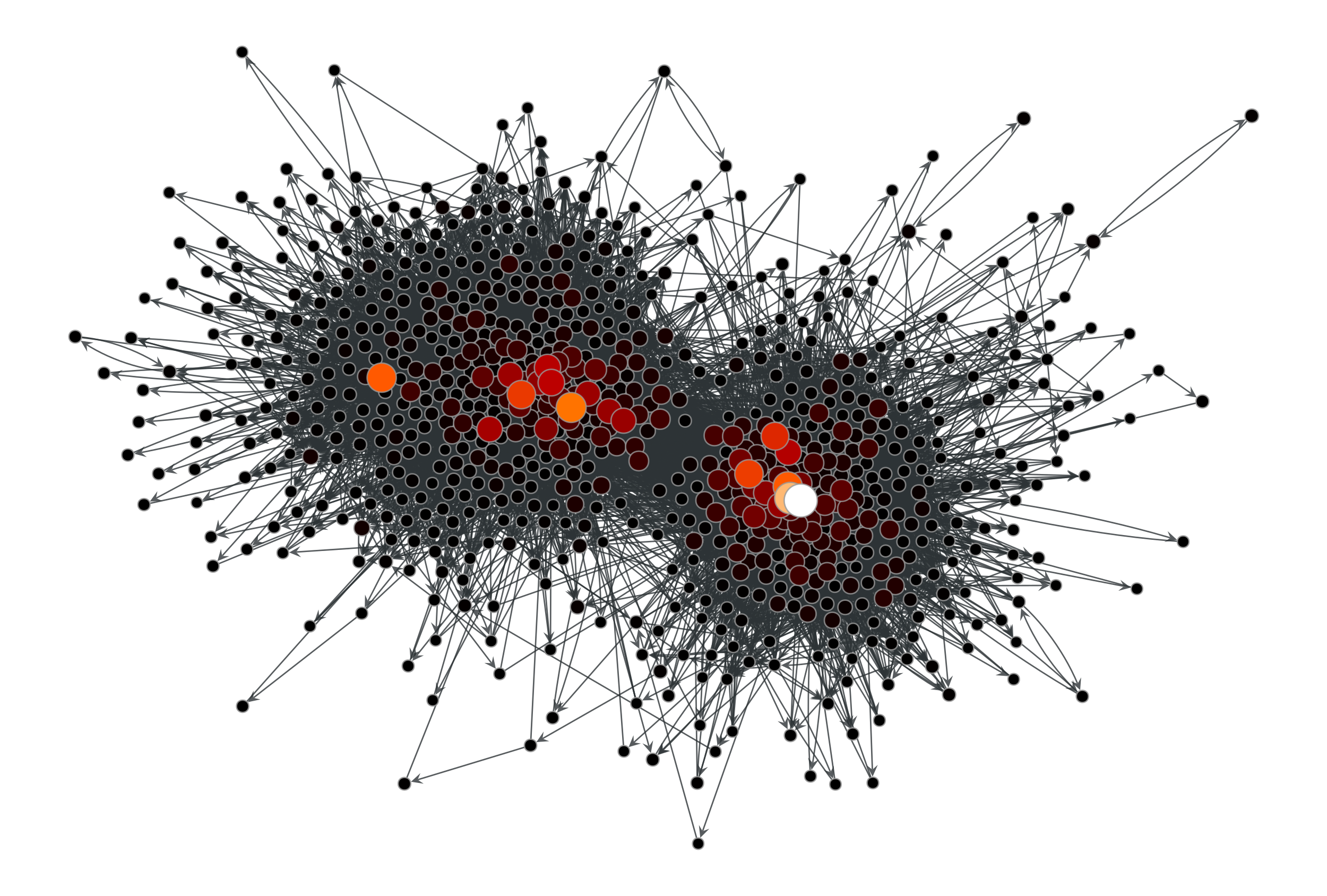PageRank values of the a political blogs network of [adamic-polblogs].

Now with a personalization vector, and edge weights:

>>> d = g.degree_property_map("total")
>>> periphery = d.a <= 2
>>> p = g.new_vertex_property("double")
>>> p.a[periphery] = 100
>>> pr = gt.pagerank(g, pers=p)
>>> gt.graph_draw(g, pos=g.vp["pos"], vertex_fill_color=pr,
...               vertex_size=gt.prop_to_size(pr, mi=5, ma=15),
...               vorder=pr, vcmap=matplotlib.cm.gist_heat,
...               output="polblogs_pr_pers.pdf")
<...>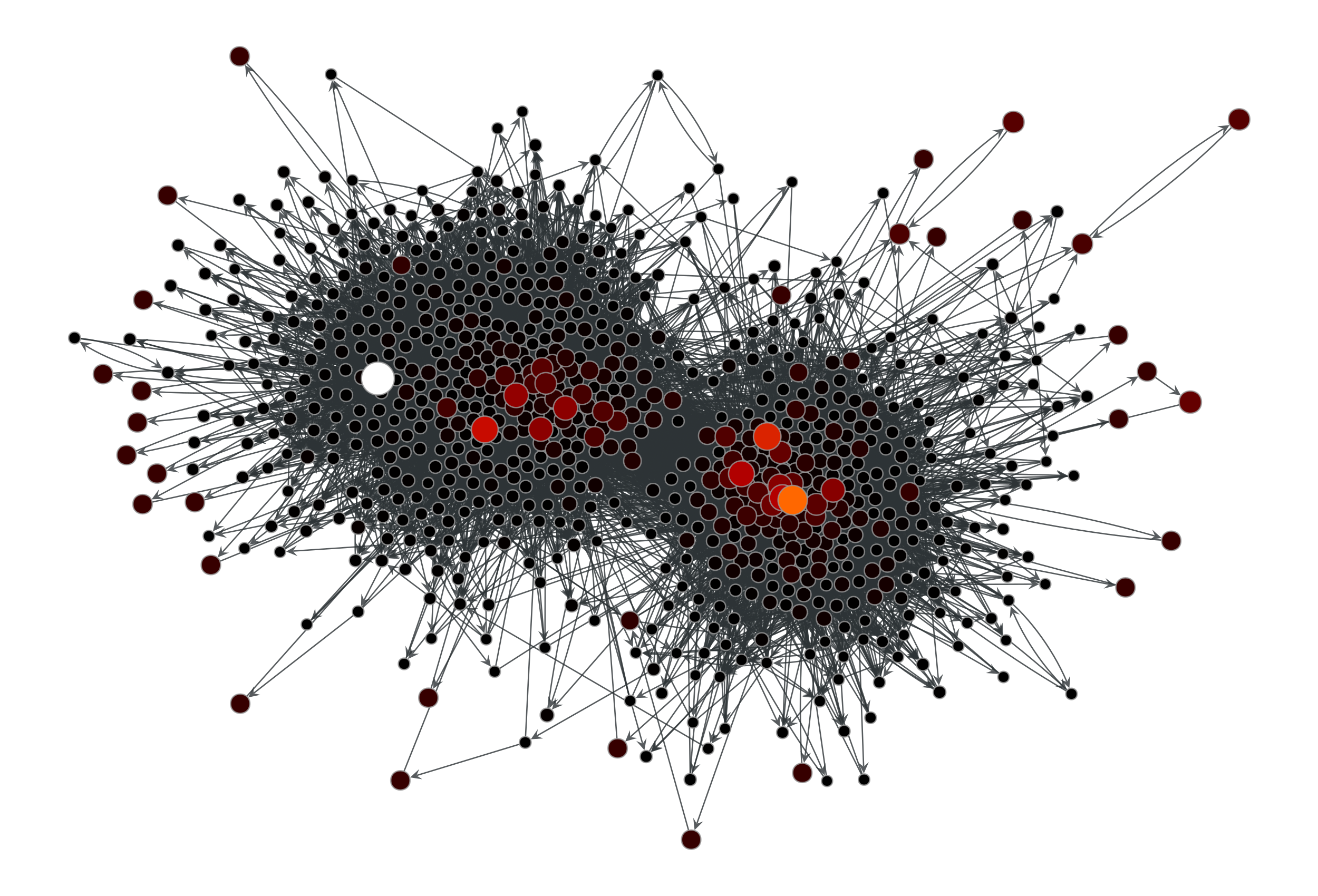Personalized PageRank values of the a political blogs network of [adamic-polblogs], where vertices with very low degree are given artificially high scores.

graph_tool.centrality.betweenness(g, pivots=None, vprop=None, eprop=None, weight=None, norm=True)[source]

Calculate the betweenness centrality for each vertex and edge.

Parameters
gGraph

Graph to be used.

pivotslist or ndarray, optional (default: None)

If provided, the betweenness will be estimated using the vertices in this list as pivots. If the list contains all nodes (the default) the algorithm will be exact, and if the vertices are randomly chosen the result will be an unbiased estimator.

vpropVertexPropertyMap, optional (default: None)

Vertex property map to store the vertex betweenness values.

epropEdgePropertyMap, optional (default: None)

Edge property map to store the edge betweenness values.

weightEdgePropertyMap, optional (default: None)

Edge property map corresponding to the weight value of each edge.

normbool, optional (default: True)

Whether or not the betweenness values should be normalized.

Returns
vertex_betweennessA vertex property map with the vertex betweenness values.
edge_betweennessAn edge property map with the edge betweenness values.

central_point_dominance

central point dominance of the graph

pagerank

PageRank centrality

eigentrust

eigentrust centrality

eigenvector

eigenvector centrality

hits

authority and hub centralities

trust_transitivity

pervasive trust transitivity

Notes

Betweenness centrality of a vertex $$C_B(v)$$ is defined as,

$C_B(v)= \sum_{s \neq v \neq t \in V \atop s \neq t} \frac{\sigma_{st}(v)}{\sigma_{st}}$

where $$\sigma_{st}$$ is the number of shortest paths from s to t, and $$\sigma_{st}(v)$$ is the number of shortest paths from s to t that pass through a vertex $$v$$. This may be normalised by dividing through the number of pairs of vertices not including v, which is $$(n-1)(n-2)/2$$, for undirected graphs, or $$(n-1)(n-2)$$ for directed ones.

The algorithm used here is defined in [brandes-faster-2001], and has a complexity of $$O(VE)$$ for unweighted graphs and $$O(VE + V(V+E)\log V)$$ for weighted graphs. The space complexity is $$O(VE)$$.

If the pivots parameter is given, the complexity will be instead $$O(PE)$$ for unweighted graphs and $$O(PE + P(V+E)\log V)$$ for weighted graphs, where $$P$$ is the number of pivot vertices.

If enabled during compilation, this algorithm runs in parallel.

References

betweenness-wikipedia

http://en.wikipedia.org/wiki/Centrality#Betweenness_centrality

brandes-faster-2001(1,2)

U. Brandes, “A faster algorithm for betweenness centrality”, Journal of Mathematical Sociology, 2001, DOI: 10.1080/0022250X.2001.9990249 [sci-hub, @tor]

brandes-centrality-2007

U. Brandes, C. Pich, “Centrality estimation in large networks”, Int. J. Bifurcation Chaos 17, 2303 (2007). DOI: 10.1142/S0218127407018403 [sci-hub, @tor]

L. A. Adamic and N. Glance, “The political blogosphere and the 2004 US Election”, in Proceedings of the WWW-2005 Workshop on the Weblogging Ecosystem (2005). DOI: 10.1145/1134271.1134277 [sci-hub, @tor]

Examples

>>> g = gt.collection.data["polblogs"]
>>> g = gt.GraphView(g, vfilt=gt.label_largest_component(g))
>>> vp, ep = gt.betweenness(g)
>>> gt.graph_draw(g, pos=g.vp["pos"], vertex_fill_color=vp,
...               vertex_size=gt.prop_to_size(vp, mi=5, ma=15),
...               edge_pen_width=gt.prop_to_size(ep, mi=0.5, ma=5),
...               vcmap=matplotlib.cm.gist_heat,
...               vorder=vp, output="polblogs_betweenness.pdf")
<...>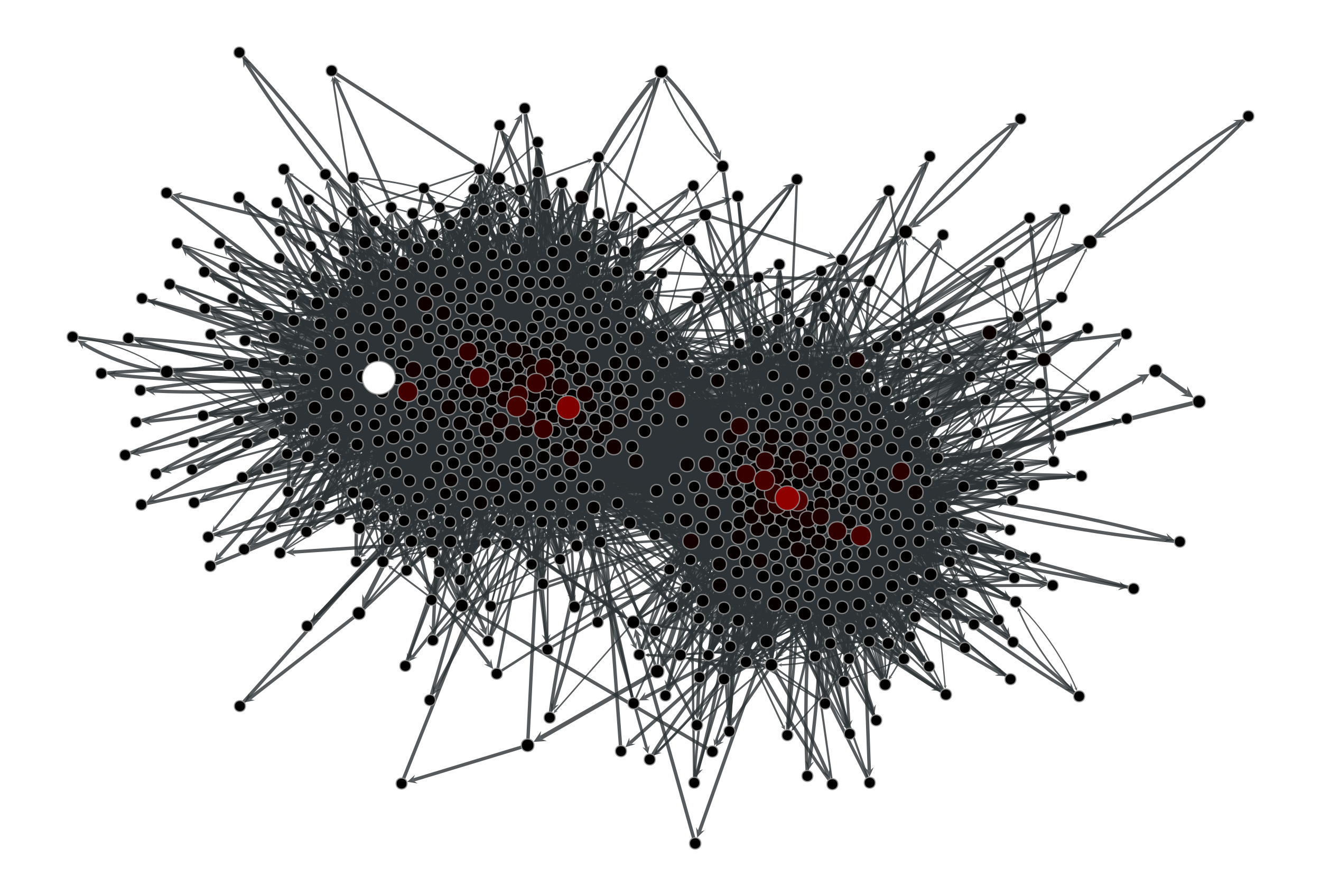Betweenness values of the a political blogs network of [adamic-polblogs].

graph_tool.centrality.closeness(g, weight=None, source=None, vprop=None, norm=True, harmonic=False)[source]

Calculate the closeness centrality for each vertex.

Parameters
gGraph

Graph to be used.

weightEdgePropertyMap, optional (default: None)

Edge property map corresponding to the weight value of each edge.

sourceVertex, optional (default: None)

If specified, the centrality is computed for this vertex alone.

vpropVertexPropertyMap, optional (default: None)

Vertex property map to store the vertex centrality values.

normbool, optional (default: True)

Whether or not the centrality values should be normalized.

harmonicbool, optional (default: False)

If true, the sum of the inverse of the distances will be computed, instead of the inverse of the sum.

Returns
vertex_closenessVertexPropertyMap

A vertex property map with the vertex closeness values.

central_point_dominance

central point dominance of the graph

pagerank

PageRank centrality

eigentrust

eigentrust centrality

eigenvector

eigenvector centrality

hits

authority and hub centralities

trust_transitivity

pervasive trust transitivity

Notes

The closeness centrality of a vertex $$i$$ is defined as,

$c_i = \frac{1}{\sum_j d_{ij}}$

where $$d_{ij}$$ is the (possibly directed and/or weighted) distance from $$i$$ to $$j$$. In case there is no path between the two vertices, here the distance is taken to be zero.

If harmonic == True, the definition becomes

$c_i = \sum_j\frac{1}{d_{ij}},$

but now, in case there is no path between the two vertices, we take $$d_{ij} \to\infty$$ such that $$1/d_{ij}=0$$.

If norm == True, the values of $$c_i$$ are normalized by $$n_i-1$$ where $$n_i$$ is the size of the (out-) component of $$i$$. If harmonic == True, they are instead simply normalized by $$V-1$$.

The algorithm complexity of $$O(V(V + E))$$ for unweighted graphs and $$O(V(V+E) \log V)$$ for weighted graphs. If the option source is specified, this drops to $$O(V + E)$$ and $$O((V+E)\log V)$$ respectively.

If enabled during compilation, this algorithm runs in parallel.

References

closeness-wikipedia

https://en.wikipedia.org/wiki/Closeness_centrality

opsahl-node-2010

Opsahl, T., Agneessens, F., Skvoretz, J., “Node centrality in weighted networks: Generalizing degree and shortest paths”. Social Networks 32, 245-251, 2010 DOI: 10.1016/j.socnet.2010.03.006 [sci-hub, @tor]

L. A. Adamic and N. Glance, “The political blogosphere and the 2004 US Election”, in Proceedings of the WWW-2005 Workshop on the Weblogging Ecosystem (2005). DOI: 10.1145/1134271.1134277 [sci-hub, @tor]

Examples

>>> g = gt.collection.data["polblogs"]
>>> g = gt.GraphView(g, vfilt=gt.label_largest_component(g))
>>> c = gt.closeness(g)
>>> gt.graph_draw(g, pos=g.vp["pos"], vertex_fill_color=c,
...               vertex_size=gt.prop_to_size(c, mi=5, ma=15),
...               vcmap=matplotlib.cm.gist_heat,
...               vorder=c, output="polblogs_closeness.pdf")
<...>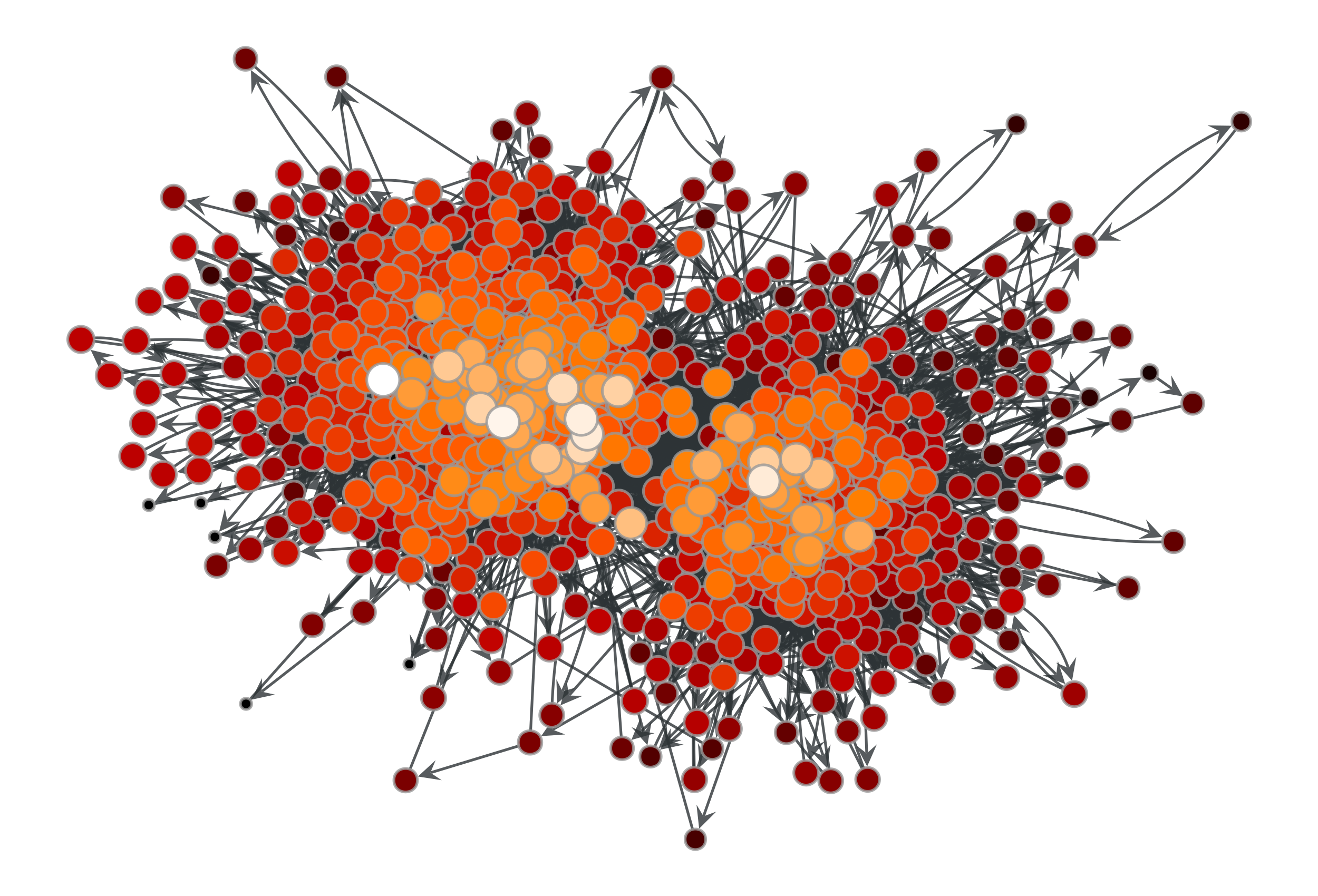Closeness values of the a political blogs network of [adamic-polblogs].

graph_tool.centrality.central_point_dominance(g, betweenness)[source]

Calculate the central point dominance of the graph, given the betweenness centrality of each vertex.

Parameters
gGraph

Graph to be used.

betweennessVertexPropertyMap

Vertex property map with the betweenness centrality values. The values must be normalized.

Returns
cpfloat

The central point dominance.

betweenness

betweenness centrality

Notes

Let $$v^*$$ be the vertex with the largest relative betweenness centrality; then, the central point dominance [freeman-set-1977] is defined as:

$C'_B = \frac{1}{|V|-1} \sum_{v} C_B(v^*) - C_B(v)$

where $$C_B(v)$$ is the normalized betweenness centrality of vertex v. The value of $$C_B$$ lies in the range [0,1].

The algorithm has a complexity of $$O(V)$$.

References

freeman-set-1977(1,2)

Linton C. Freeman, “A Set of Measures of Centrality Based on Betweenness”, Sociometry, Vol. 40, No. 1, pp. 35-41, 1977, DOI: 10.2307/3033543 [sci-hub, @tor]

Examples

>>> g = gt.collection.data["polblogs"]
>>> g = gt.GraphView(g, vfilt=gt.label_largest_component(g))
>>> vp, ep = gt.betweenness(g)
>>> print(gt.central_point_dominance(g, vp))
0.105683...
graph_tool.centrality.eigenvector(g, weight=None, vprop=None, epsilon=1e-06, max_iter=None)[source]

Calculate the eigenvector centrality of each vertex in the graph, as well as the largest eigenvalue.

Parameters
gGraph

Graph to be used.

weightEdgePropertyMap (optional, default: None)

Edge property map with the edge weights.

vpropVertexPropertyMap, optional (default: None)

Vertex property map where the values of eigenvector must be stored. If provided, it will be used uninitialized.

epsilonfloat, optional (default: 1e-6)

Convergence condition. The iteration will stop if the total delta of all vertices are below this value.

max_iterint, optional (default: None)

If supplied, this will limit the total number of iterations.

Returns
eigenvaluefloat

The largest eigenvalue of the (weighted) adjacency matrix.

eigenvectorVertexPropertyMap

A vertex property map containing the eigenvector values.

betweenness

betweenness centrality

pagerank

PageRank centrality

hits

authority and hub centralities

trust_transitivity

pervasive trust transitivity

Notes

The eigenvector centrality $$\mathbf{x}$$ is the eigenvector of the (weighted) adjacency matrix with the largest eigenvalue $$\lambda$$, i.e. it is the solution of

$\mathbf{A}\mathbf{x} = \lambda\mathbf{x},$

where $$\mathbf{A}$$ is the (weighted) adjacency matrix and $$\lambda$$ is the largest eigenvalue.

The algorithm uses the power method which has a topology-dependent complexity of $$O\left(N\times\frac{-\log\epsilon}{\log|\lambda_1/\lambda_2|}\right)$$, where $$N$$ is the number of vertices, $$\epsilon$$ is the epsilon parameter, and $$\lambda_1$$ and $$\lambda_2$$ are the largest and second largest eigenvalues of the (weighted) adjacency matrix, respectively.

If enabled during compilation, this algorithm runs in parallel.

References

eigenvector-centrality

http://en.wikipedia.org/wiki/Centrality#Eigenvector_centrality

power-method

http://en.wikipedia.org/wiki/Power_iteration

langville-survey-2005

A. N. Langville, C. D. Meyer, “A Survey of Eigenvector Methods for Web Information Retrieval”, SIAM Review, vol. 47, no. 1, pp. 135-161, 2005, DOI: 10.1137/S0036144503424786 [sci-hub, @tor]

L. A. Adamic and N. Glance, “The political blogosphere and the 2004 US Election”, in Proceedings of the WWW-2005 Workshop on the Weblogging Ecosystem (2005). DOI: 10.1145/1134271.1134277 [sci-hub, @tor]

Examples

>>> g = gt.collection.data["polblogs"]
>>> g = gt.GraphView(g, vfilt=gt.label_largest_component(g))
>>> w = g.new_edge_property("double")
>>> w.a = np.random.random(len(w.a)) * 42
>>> ee, x = gt.eigenvector(g, w)
>>> gt.graph_draw(g, pos=g.vp["pos"], vertex_fill_color=x,
...               vertex_size=gt.prop_to_size(x, mi=5, ma=15),
...               vcmap=matplotlib.cm.gist_heat,
...               vorder=x, output="polblogs_eigenvector.pdf")
<...>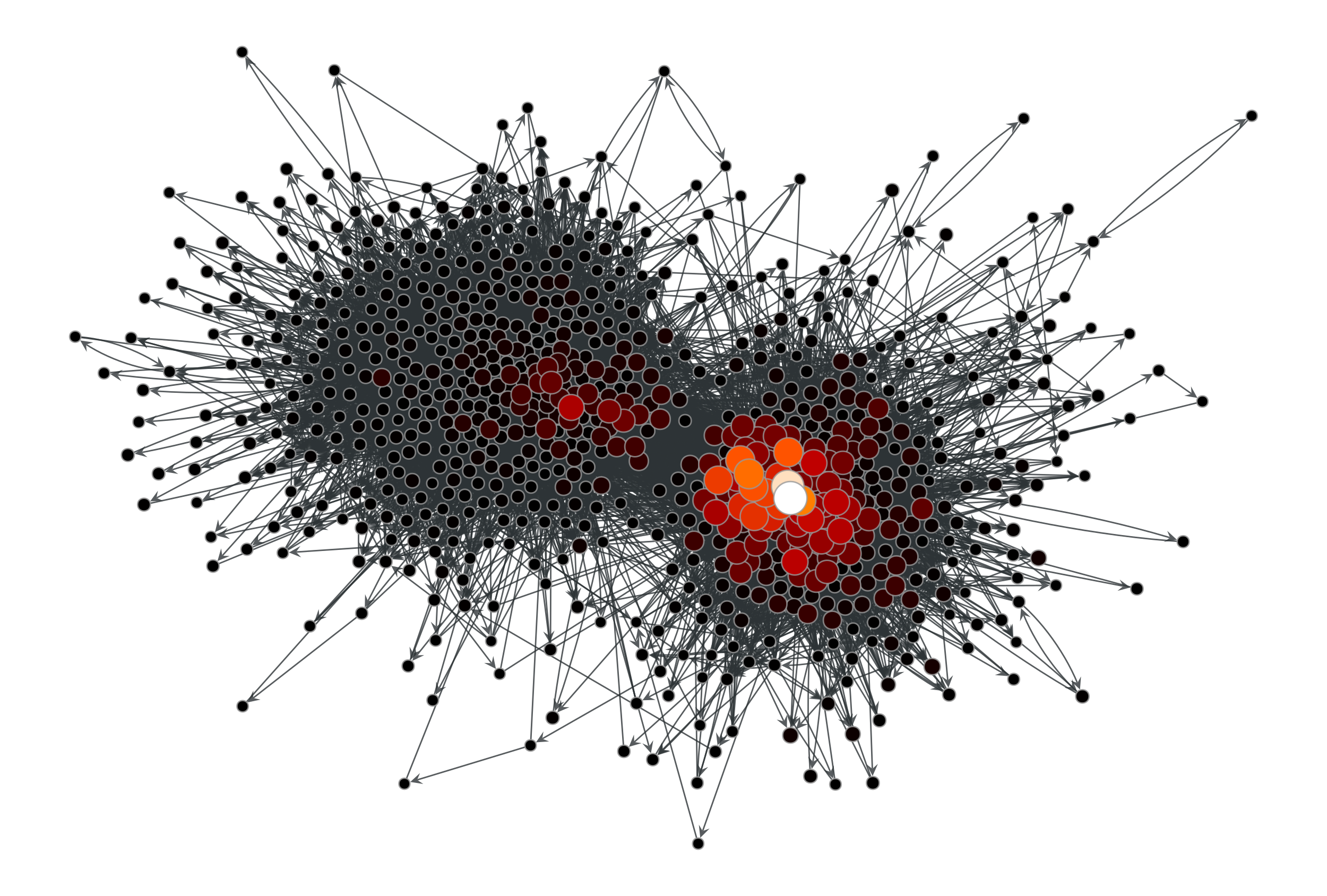Eigenvector values of the a political blogs network of [adamic-polblogs], with random weights attributed to the edges.

graph_tool.centrality.katz(g, alpha=0.01, beta=None, weight=None, vprop=None, epsilon=1e-06, max_iter=None, norm=True)[source]

Calculate the Katz centrality of each vertex in the graph.

Parameters
gGraph

Graph to be used.

weightEdgePropertyMap (optional, default: None)

Edge property map with the edge weights.

alphafloat, optional (default: 0.01)

Free parameter $$\alpha$$. This must be smaller than the inverse of the largest eigenvalue of the adjacency matrix.

betaVertexPropertyMap, optional (default: None)

Vertex property map where the local personalization values. If not provided, the global value of 1 will be used.

vpropVertexPropertyMap, optional (default: None)

Vertex property map where the values of eigenvector must be stored. If provided, it will be used uninitialized.

epsilonfloat, optional (default: 1e-6)

Convergence condition. The iteration will stop if the total delta of all vertices are below this value.

max_iterint, optional (default: None)

If supplied, this will limit the total number of iterations.

normbool, optional (default: True)

Whether or not the centrality values should be normalized.

Returns
centralityVertexPropertyMap

A vertex property map containing the Katz centrality values.

betweenness

betweenness centrality

pagerank

PageRank centrality

eigenvector

eigenvector centrality

hits

authority and hub centralities

trust_transitivity

pervasive trust transitivity

Notes

The Katz centrality $$\mathbf{x}$$ is the solution of the nonhomogeneous linear system

$\mathbf{x} = \alpha\mathbf{A}\mathbf{x} + \mathbf{\beta},$

where $$\mathbf{A}$$ is the (weighted) adjacency matrix and $$\mathbf{\beta}$$ is the personalization vector (if not supplied, $$\mathbf{\beta} = \mathbf{1}$$ is assumed).

The algorithm uses successive iterations of the equation above, which has a topology-dependent convergence complexity.

If enabled during compilation, this algorithm runs in parallel.

References

katz-centrality

http://en.wikipedia.org/wiki/Katz_centrality

katz-new

L. Katz, “A new status index derived from sociometric analysis”, Psychometrika 18, Number 1, 39-43, 1953, DOI: 10.1007/BF02289026 [sci-hub, @tor]

L. A. Adamic and N. Glance, “The political blogosphere and the 2004 US Election”, in Proceedings of the WWW-2005 Workshop on the Weblogging Ecosystem (2005). DOI: 10.1145/1134271.1134277 [sci-hub, @tor]

Examples

>>> g = gt.collection.data["polblogs"]
>>> g = gt.GraphView(g, vfilt=gt.label_largest_component(g))
>>> w = g.new_edge_property("double")
>>> w.a = np.random.random(len(w.a))
>>> x = gt.katz(g, weight=w)
>>> gt.graph_draw(g, pos=g.vp["pos"], vertex_fill_color=x,
...               vertex_size=gt.prop_to_size(x, mi=5, ma=15),
...               vcmap=matplotlib.cm.gist_heat,
...               vorder=x, output="polblogs_katz.pdf")
<...>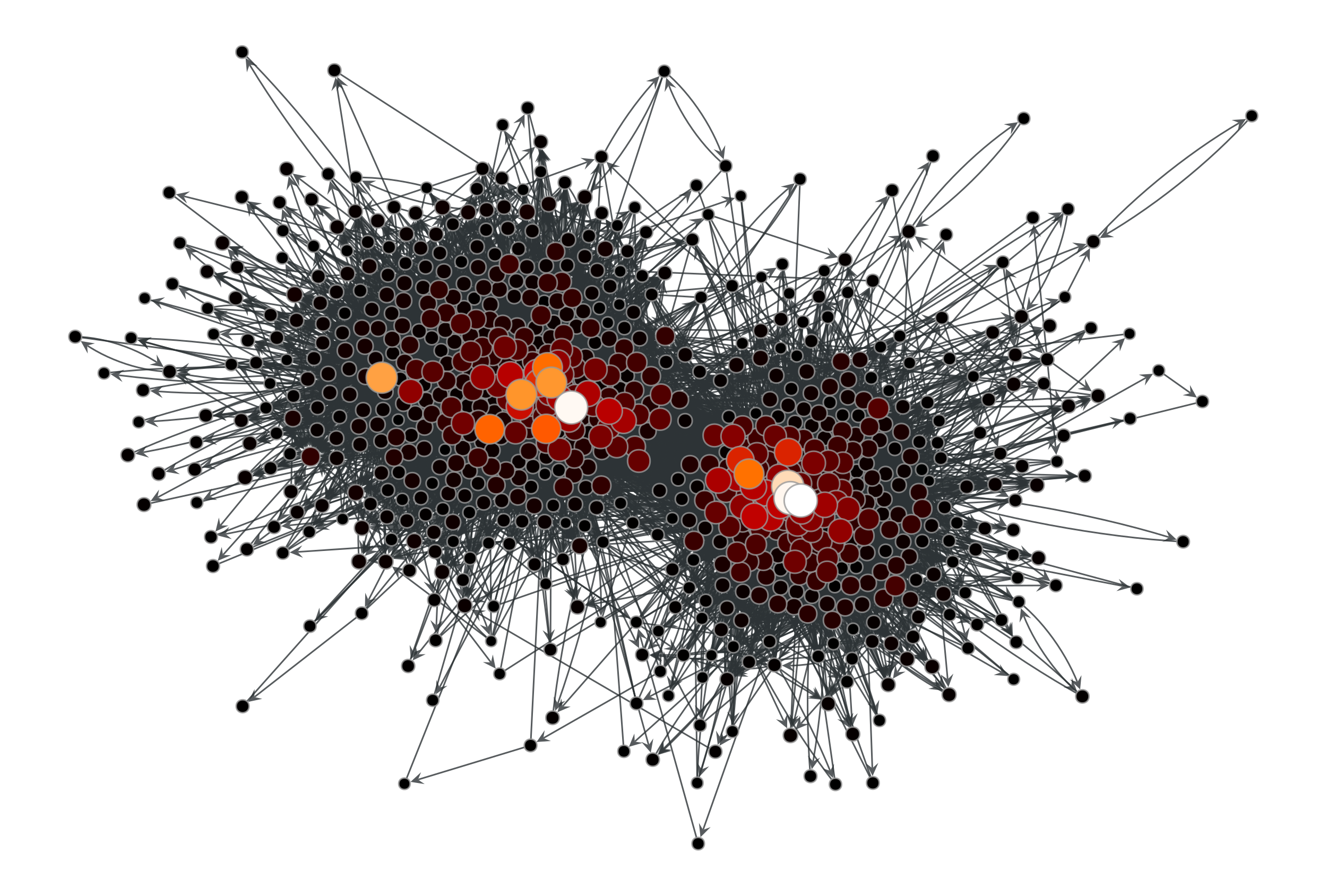Katz centrality values of the a political blogs network of [adamic-polblogs], with random weights attributed to the edges.

graph_tool.centrality.hits(g, weight=None, xprop=None, yprop=None, epsilon=1e-06, max_iter=None)[source]

Calculate the authority and hub centralities of each vertex in the graph.

Parameters
gGraph

Graph to be used.

weightEdgePropertyMap (optional, default: None)

Edge property map with the edge weights.

xpropVertexPropertyMap, optional (default: None)

Vertex property map where the authority centrality must be stored.

ypropVertexPropertyMap, optional (default: None)

Vertex property map where the hub centrality must be stored.

epsilonfloat, optional (default: 1e-6)

Convergence condition. The iteration will stop if the total delta of all vertices are below this value.

max_iterint, optional (default: None)

If supplied, this will limit the total number of iterations.

Returns
eigfloat

The largest eigenvalue of the cocitation matrix.

xVertexPropertyMap

A vertex property map containing the authority centrality values.

yVertexPropertyMap

A vertex property map containing the hub centrality values.

betweenness

betweenness centrality

eigenvector

eigenvector centrality

pagerank

PageRank centrality

trust_transitivity

pervasive trust transitivity

Notes

The Hyperlink-Induced Topic Search (HITS) centrality assigns hub ($$\mathbf{y}$$) and authority ($$\mathbf{x}$$) centralities to the vertices, following:

\begin{split}\begin{align} \mathbf{x} &= \alpha\mathbf{A}\mathbf{y} \\ \mathbf{y} &= \beta\mathbf{A}^T\mathbf{x} \end{align}\end{split}

where $$\mathbf{A}$$ is the (weighted) adjacency matrix and $$\lambda = 1/(\alpha\beta)$$ is the largest eigenvalue of the cocitation matrix, $$\mathbf{A}\mathbf{A}^T$$. (Without loss of generality, we set $$\beta=1$$ in the algorithm.)

The algorithm uses the power method which has a topology-dependent complexity of $$O\left(N\times\frac{-\log\epsilon}{\log|\lambda_1/\lambda_2|}\right)$$, where $$N$$ is the number of vertices, $$\epsilon$$ is the epsilon parameter, and $$\lambda_1$$ and $$\lambda_2$$ are the largest and second largest eigenvalues of the (weighted) cocitation matrix, respectively.

If enabled during compilation, this algorithm runs in parallel.

References

hits-algorithm

http://en.wikipedia.org/wiki/HITS_algorithm

kleinberg-authoritative

J. Kleinberg, “Authoritative sources in a hyperlinked environment”, Journal of the ACM 46 (5): 604-632, 1999, DOI: 10.1145/324133.324140 [sci-hub, @tor].

power-method

http://en.wikipedia.org/wiki/Power_iteration

L. A. Adamic and N. Glance, “The political blogosphere and the 2004 US Election”, in Proceedings of the WWW-2005 Workshop on the Weblogging Ecosystem (2005). DOI: 10.1145/1134271.1134277 [sci-hub, @tor]

Examples

>>> g = gt.collection.data["polblogs"]
>>> g = gt.GraphView(g, vfilt=gt.label_largest_component(g))
>>> ee, x, y = gt.hits(g)
>>> gt.graph_draw(g, pos=g.vp["pos"], vertex_fill_color=x,
...               vertex_size=gt.prop_to_size(x, mi=5, ma=15),
...               vcmap=matplotlib.cm.gist_heat,
...               vorder=x, output="polblogs_hits_auths.pdf")
<...>
>>> gt.graph_draw(g, pos=g.vp["pos"], vertex_fill_color=y,
...               vertex_size=gt.prop_to_size(y, mi=5, ma=15),
...               vcmap=matplotlib.cm.gist_heat,
...               vorder=y, output="polblogs_hits_hubs.pdf")
<...>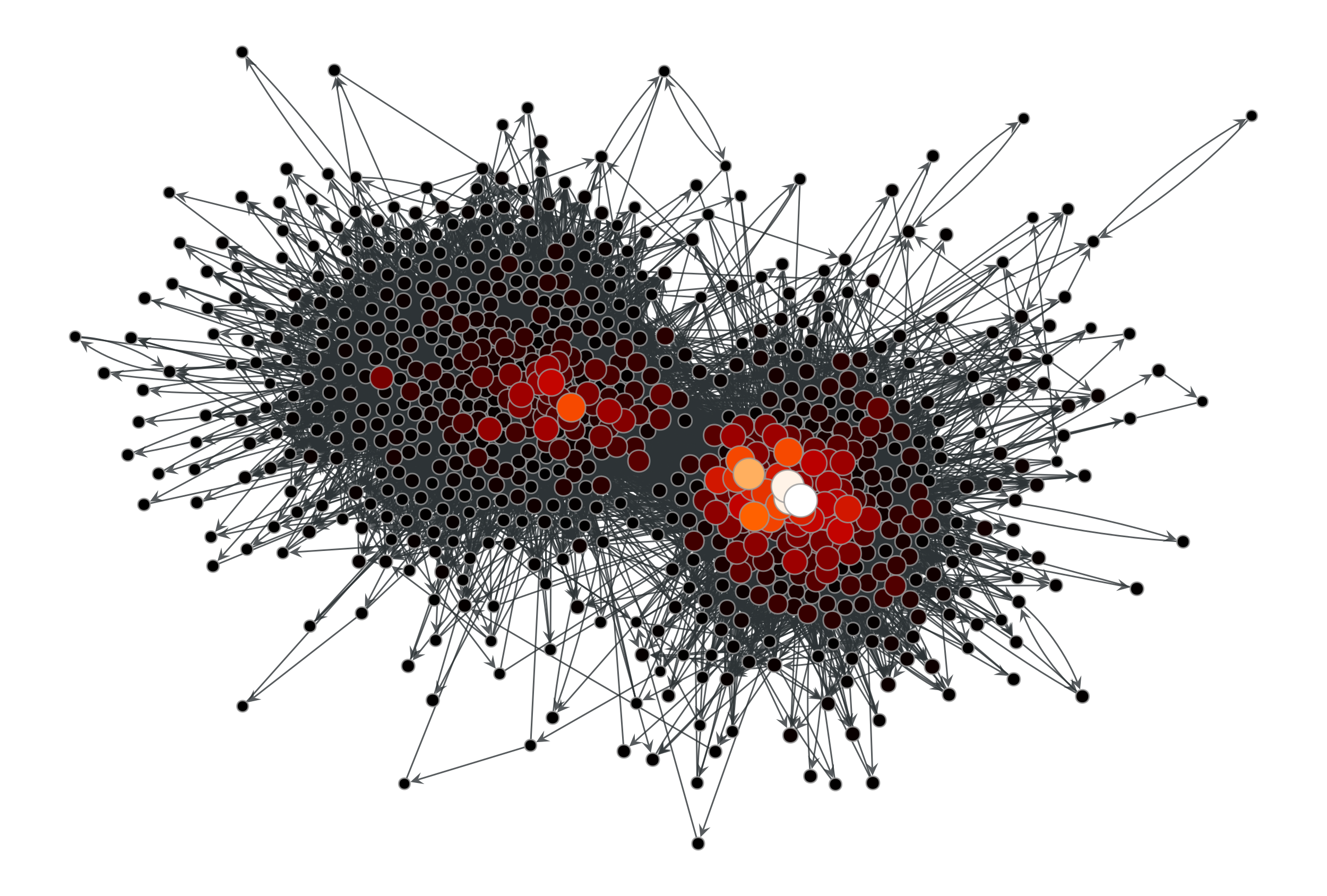HITS authority values of the a political blogs network of [adamic-polblogs].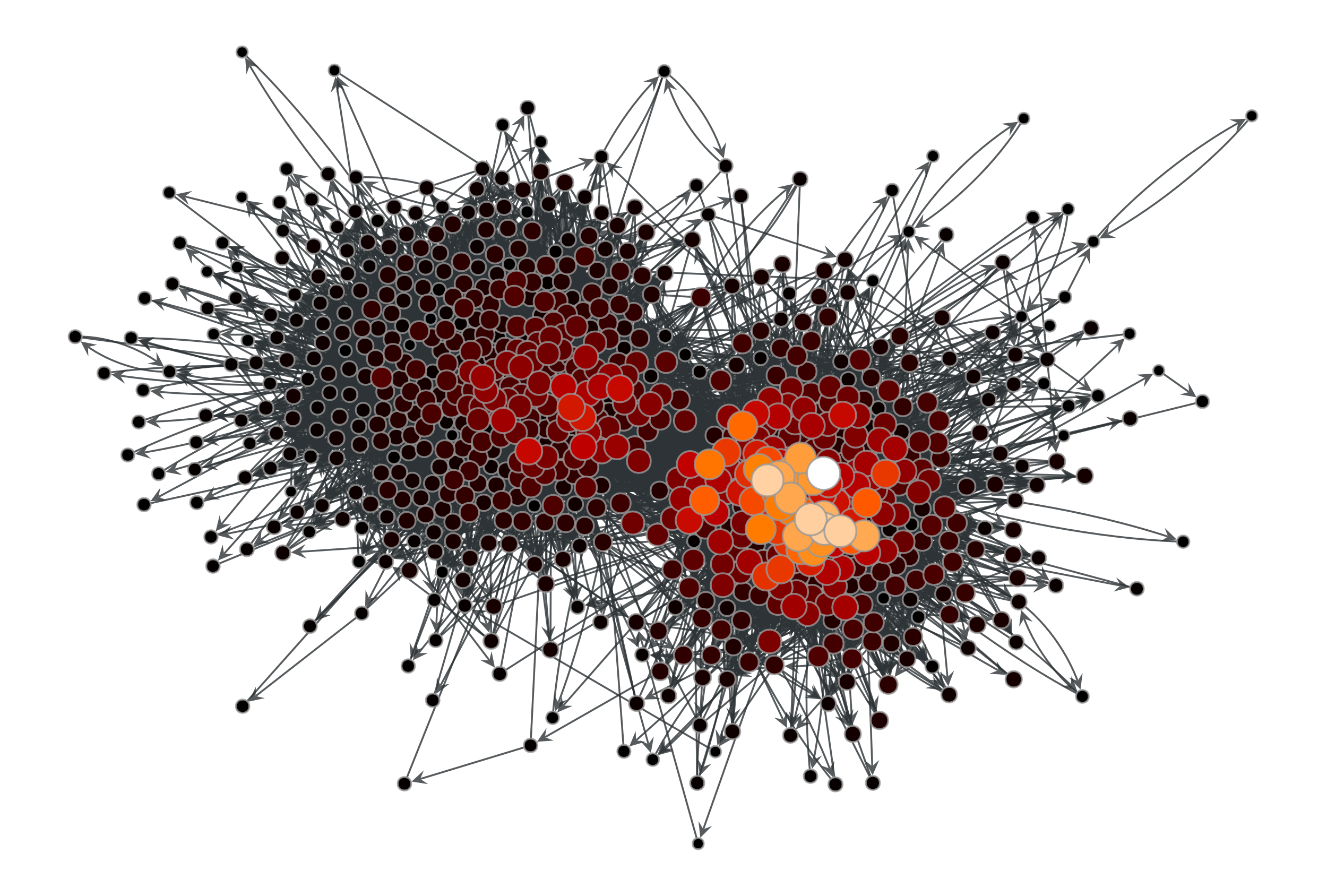HITS hub values of the a political blogs network of [adamic-polblogs].

graph_tool.centrality.eigentrust(g, trust_map, vprop=None, norm=False, epsilon=1e-06, max_iter=0, ret_iter=False)[source]

Calculate the eigentrust centrality of each vertex in the graph.

Parameters
gGraph

Graph to be used.

trust_mapEdgePropertyMap

Edge property map with the values of trust associated with each edge. The values must lie in the range [0,1].

vpropVertexPropertyMap, optional (default: None)

Vertex property map where the values of eigentrust must be stored.

normbool, optional (default: False)

Norm eigentrust values so that the total sum equals 1.

epsilonfloat, optional (default: 1e-6)

Convergence condition. The iteration will stop if the total delta of all vertices are below this value.

max_iterint, optional (default: None)

If supplied, this will limit the total number of iterations.

ret_iterbool, optional (default: False)

If true, the total number of iterations is also returned.

Returns
eigentrustVertexPropertyMap

A vertex property map containing the eigentrust values.

betweenness

betweenness centrality

pagerank

PageRank centrality

trust_transitivity

pervasive trust transitivity

Notes

The eigentrust [kamvar-eigentrust-2003] values $$t_i$$ correspond the following limit

$\mathbf{t} = \lim_{n\to\infty} \left(C^T\right)^n \mathbf{c}$

where $$c_i = 1/|V|$$ and the elements of the matrix $$C$$ are the normalized trust values:

$c_{ij} = \frac{\max(s_{ij},0)}{\sum_{j} \max(s_{ij}, 0)}$

The algorithm has a topology-dependent complexity.

If enabled during compilation, this algorithm runs in parallel.

References

kamvar-eigentrust-2003(1,2)

S. D. Kamvar, M. T. Schlosser, H. Garcia-Molina “The eigentrust algorithm for reputation management in p2p networks”, Proceedings of the 12th international conference on World Wide Web, Pages: 640 - 651, 2003, DOI: 10.1145/775152.775242 [sci-hub, @tor]

L. A. Adamic and N. Glance, “The political blogosphere and the 2004 US Election”, in Proceedings of the WWW-2005 Workshop on the Weblogging Ecosystem (2005). DOI: 10.1145/1134271.1134277 [sci-hub, @tor]

Examples

>>> g = gt.collection.data["polblogs"]
>>> g = gt.GraphView(g, vfilt=gt.label_largest_component(g))
>>> w = g.new_edge_property("double")
>>> w.a = np.random.random(len(w.a)) * 42
>>> t = gt.eigentrust(g, w)
>>> gt.graph_draw(g, pos=g.vp["pos"], vertex_fill_color=t,
...               vertex_size=gt.prop_to_size(t, mi=5, ma=15),
...               vcmap=matplotlib.cm.gist_heat,
...               vorder=t, output="polblogs_eigentrust.pdf")
<...>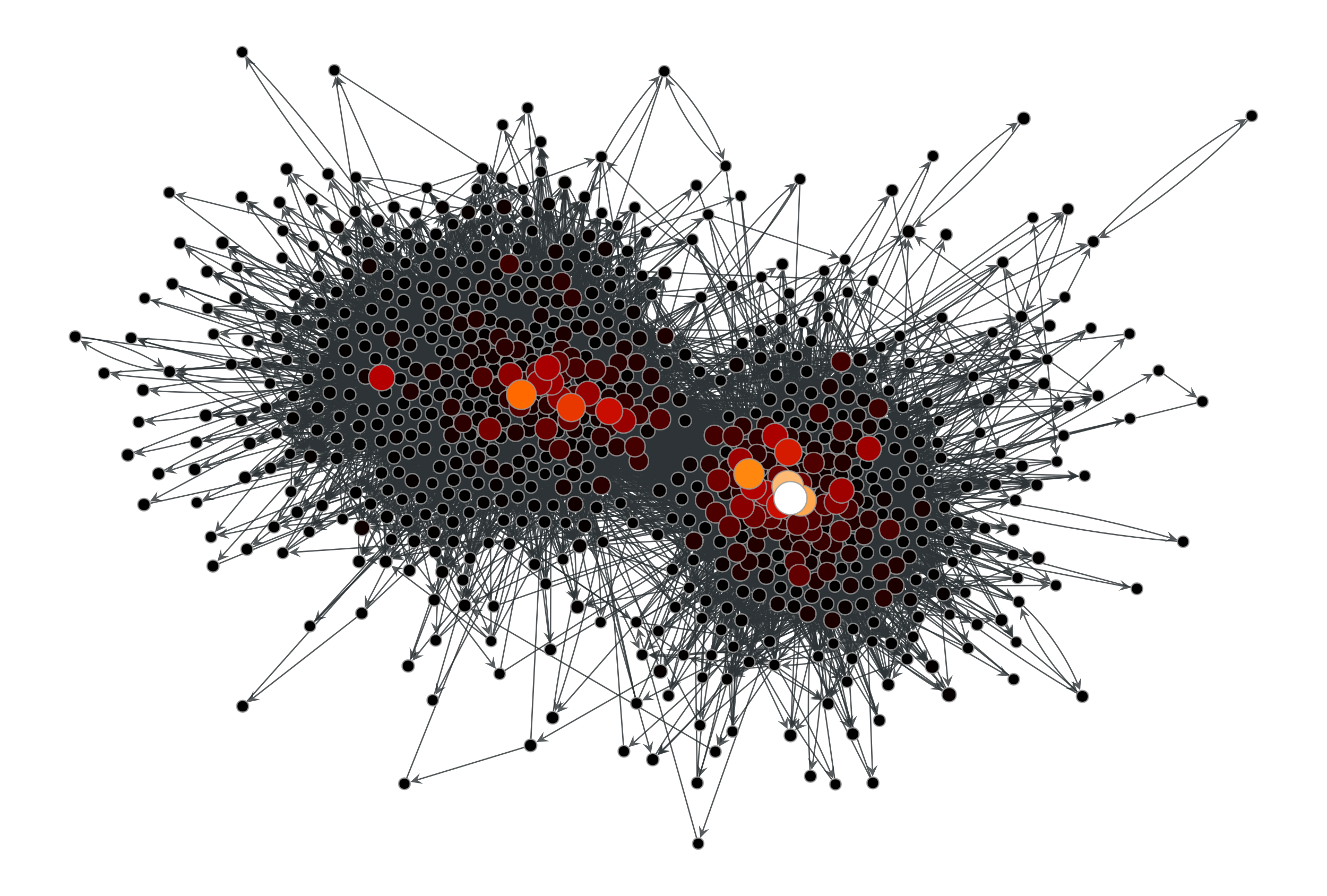Eigentrust values of the a political blogs network of [adamic-polblogs], with random weights attributed to the edges.

graph_tool.centrality.trust_transitivity(g, trust_map, source=None, target=None, vprop=None)[source]

Calculate the pervasive trust transitivity between chosen (or all) vertices in the graph.

Parameters
gGraph

Graph to be used.

trust_mapEdgePropertyMap

Edge property map with the values of trust associated with each edge. The values must lie in the range [0,1].

sourceVertex (optional, default: None)

Source vertex. All trust values are computed relative to this vertex. If left unspecified, the trust values for all sources are computed.

targetVertex (optional, default: None)

The only target for which the trust value will be calculated. If left unspecified, the trust values for all targets are computed.

vpropVertexPropertyMap (optional, default: None)

A vertex property map where the values of transitive trust must be stored.

Returns
trust_transitivityVertexPropertyMap or float

A vertex vector property map containing, for each source vertex, a vector with the trust values for the other vertices. If only one of source or target is specified, this will be a single-valued vertex property map containing the trust vector from/to the source/target vertex to/from the rest of the network. If both source and target are specified, the result is a single float, with the corresponding trust value for the target.

eigentrust

eigentrust centrality

betweenness

betweenness centrality

pagerank

PageRank centrality

Notes

The pervasive trust transitivity between vertices i and j is defined as

$t_{ij} = \frac{\sum_m A_{m,j} w^2_{G\setminus\{j\}}(i\to m)c_{m,j}} {\sum_m A_{m,j} w_{G\setminus\{j\}}(i\to m)}$

where $$A_{ij}$$ is the adjacency matrix, $$c_{ij}$$ is the direct trust from i to j, and $$w_G(i\to j)$$ is the weight of the path with maximum weight from i to j, computed as

$w_G(i\to j) = \prod_{e\in i\to j} c_e.$

The algorithm measures the transitive trust by finding the paths with maximum weight, using Dijkstra’s algorithm, to all in-neighbors of a given target. This search needs to be performed repeatedly for every target, since it needs to be removed from the graph first. For each given source, the resulting complexity is therefore $$O(V^2\log V)$$ for all targets, and $$O(V\log V)$$ for a single target. For a given target, the complexity for obtaining the trust from all given sources is $$O(kV\log V)$$, where $$k$$ is the in-degree of the target. Thus, the complexity for obtaining the complete trust matrix is $$O(EV\log V)$$, where $$E$$ is the number of edges in the network.

If enabled during compilation, this algorithm runs in parallel.

References

richters-trust-2010

Oliver Richters and Tiago P. Peixoto, “Trust Transitivity in Social Networks,” PLoS ONE 6, no. 4: e1838 (2011), DOI: 10.1371/journal.pone.0018384 [sci-hub, @tor]

L. A. Adamic and N. Glance, “The political blogosphere and the 2004 US Election”, in Proceedings of the WWW-2005 Workshop on the Weblogging Ecosystem (2005). DOI: 10.1145/1134271.1134277 [sci-hub, @tor]

Examples

>>> g = gt.collection.data["polblogs"]
>>> g = gt.GraphView(g, vfilt=gt.label_largest_component(g))
>>> g = gt.Graph(g, prune=True)
>>> w = g.new_edge_property("double")
>>> w.a = np.random.random(len(w.a))
>>> t = gt.trust_transitivity(g, w, source=g.vertex(42))
>>> gt.graph_draw(g, pos=g.vp["pos"], vertex_fill_color=t,
...               vertex_size=gt.prop_to_size(t, mi=5, ma=15),
...               vcmap=matplotlib.cm.gist_heat,
...               vorder=t, output="polblogs_trust_transitivity.pdf")
<...>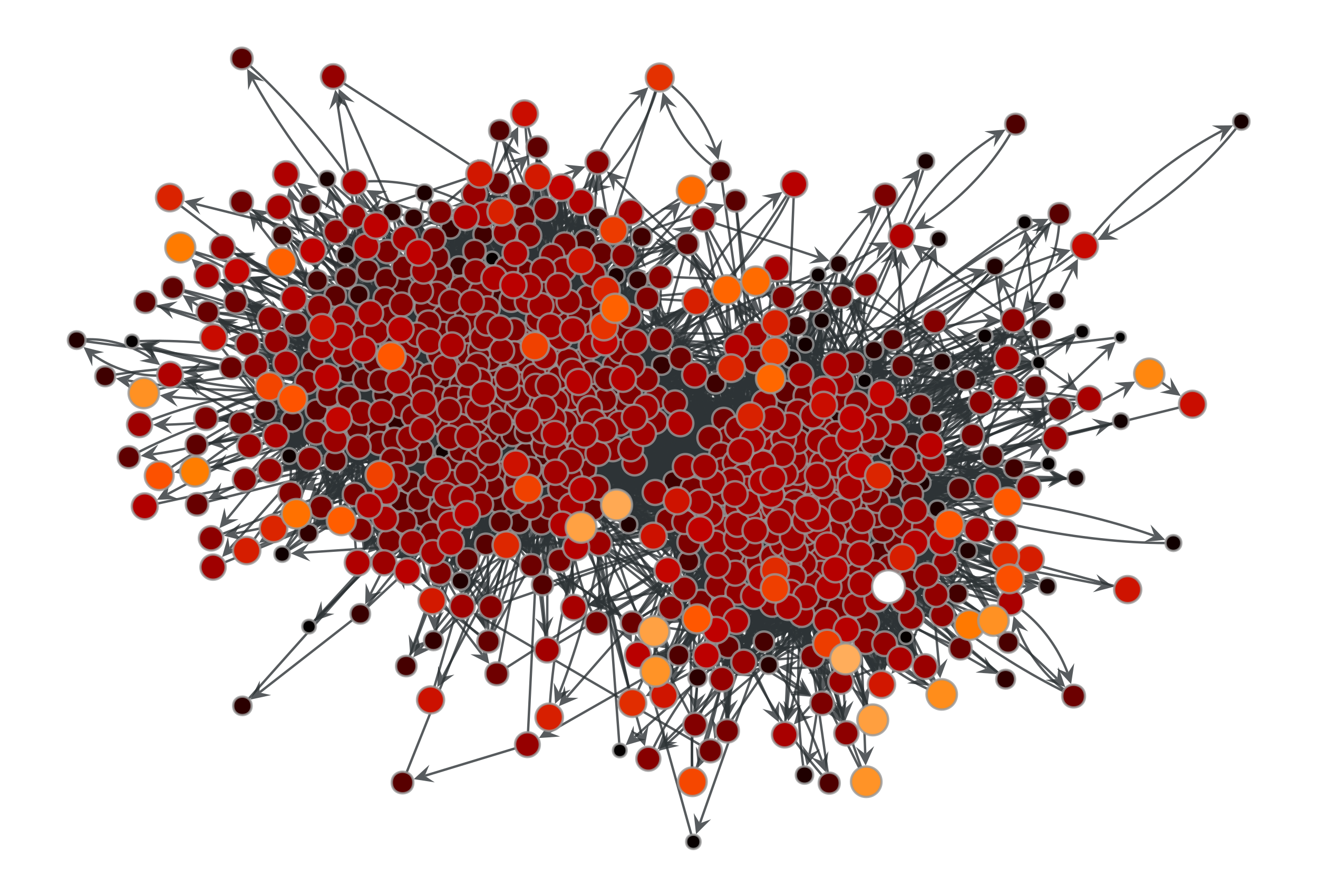Trust transitivity values from source vertex 42 of the a political blogs network of [adamic-polblogs], with random weights attributed to the edges.